# Single-shot simulations of dynamic quantum many-body systems

## Abstract

Single experimental shots of ultracold quantum gases sample the many-particle probability distribution. In a few cases such single shots could be successfully simulated from a given many-body wavefunction1,2,3,4, but for realistic time-dependent many-body dynamics this has been difficult to achieve. Here, we show how single shots can be simulated from numerical solutions of the time-dependent many-body Schrödinger equation. Using this approach, we provide first-principle explanations for fluctuations in the collision of attractive Bose–Einstein condensates (BECs), for the appearance of randomly fluctuating vortices and for the centre-of-mass fluctuations of attractive BECs in a harmonic trap. We also show how such simulations provide full counting distributions and correlation functions of any order. Such calculations have not been previously possible and our method is broadly applicable to many-body systems whose phenomenology is driven by information beyond what is typically available in low-order correlation functions.

## Main

A postulate of quantum mechanics states that the positions r1, …, rN of N particles measured in an experiment are distributed according to the N-particle probability density P(r1, …, rN) = |Ψ(r1, …, rN)|2, where Ψ(r1, …, rN) is the many-body wavefunction of the system. In many experiments the positions of individual particles cannot be measured directly. Ultracold atom experiments provide a rare exception to this rule, which is why we focus on ultracold atoms in the following, but the concept is completely general. If Ψ(r1, …, rN) is known, single experimental shots can be simulated by drawing the positions of all particles from P(r1, …, rN), which results in a vector of positions (r1′, …, rN′) that we refer to as a single shot. This has been realized for time-invariant many-body systems1,2,3,4. However, for time-dependent many-body systems it has remained a challenge. The difficulty stems from the fact that the functional form of the wavefunction is generally not known in many-body dynamics.

Attempts at simulating single shots have been reported in the context of semiclassical dynamics: several authors have interpreted classical trajectories obtained within the truncated Wigner approximation as individual realizations of experiments5,6. Under the strict condition that the Wigner function is non-negative, some authors consider this interpretation plausible7 or have fewer objections to it8. Here we show that this interpretation must also be dismissed for positive Wigner functions (see Supplementary Information). Although quantum Monte Carlo algorithms9 sample the N-particle probability to obtain lower ground state energies, for time-dependent many-body systems not even the nodal structure of the wavefunction is known in advance, and hence quantum Monte Carlo methods are less suited. For further details see Supplementary Information.

For sampling P(r1, …, rN) it helps to realize thatwhere, for example, P(r2|r1) denotes the conditional probability to find a particle at r2 given that another one is at r1. First, r1′ is drawn from P(r1), then r2′ from P(r2|r1′), then r3′ from P(r3|r2′, r1′) and so on. Note that a histogram of a single shot (r1′, …, rN′) is analogous to an image obtained in an experiment. Here we provide an algorithm to simulate single shots from any N-boson wavefunction |Ψ〉 = ∑ nCn|n〉, where |n〉 = |n1, …, nM〉 are configurations constructed by distributing N bosons over M orbitals φi (see Methods and Supplementary Information). In the following we show how single-shot simulations provide insights into strongly correlated many-body systems. The wavefunctions are obtained by numerically solving the time-dependent many-body Schrödinger equation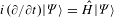using the multiconfigurational time-dependent Hartree method for bosons (MCTDHB; refs 10,11,12). Here,denotes a general many-body Hamiltonian in D dimensions with an external potential V (r) and a regularized contact interaction δε(r) = (2πε2)D/2$e - r 2 / 2 ε 2$. The mean-field parameter λ = λ0(N − 1) characterizes the interaction strength (see Methods).

We briefly recall that a BEC is condensed if its reduced single-particle density matrix has one non-zero eigenvalue of order N (ref. 13). The eigenvalues ρ1ρ2 ≥ … are known as natural occupations, the eigenvectors as natural orbitals. A BEC is fragmented if more than one natural occupation is of order N (ref. 14) (see Methods). Fully condensed states, that is, states with ρ1 = N, are of the form φ(r1)φ(r2) × × φ(rN). All particle detections are then independent of each other, because every conditional probability in equation (1) is given by |φ(r)|2. Single shots of such states merely reproduce the single-particle density ρ(r) = N|φ(r)|2. Gross–Pitaevskii (GP) mean-field states are of this form. For any other type of state, each conditional probability in (1) depends on the values of the previously detected particles and single shots do not reproduce the single-particle density.

As a first example we investigate a collision between independent, attractively interacting condensates that collide in D = 2 spatial dimensions—that is, r = (x, y) in an elongated trap V (r) with a flat bottom (see Methods for details of the trap). On the GP mean-field level, such BECs are known to pass through or bounce off each other, depending on the value of the relative phase15. GP mean-field theory assumes the many-body state to be fully condensed at all times. However, for experimentally relevant strongly interacting states, the mean-field description is fundamentally inconsistent: interactions erode the structure of the ansatz state on a timescale which is fast compared to the collision time; fully condensed attractive BECs that are spread out over large distances are not stable and fragment quickly16. We therefore choose a more stable initial state consisting of two independently created attractive BECs of 50 bosons each. It is impossible to define a relative phase for such BECs (ref. 17). Specifically, we use λ = −5.94 as an interaction strength, which is about 2% above the threshold for collapse of the ground state of all N = 100 bosons. The initial state is prepared by computing the many-body ground state of 50 bosons in the trap using M = 2 orbitals and imaginary time propagation. A copy of the ground state is placed off-centre at r = (−19.8,0). The resulting initial state is fragmented with natural occupations ρ1/N = ρ2/N = 49.4%, ρ3/N = ρ4/N = 0.6%, and is subsequently propagated in time.

Figure 1a shows the single-particle density at different times. The condensates approach each other without spreading significantly, collide and separate again. During the collision the single-particle density exhibits two maxima, such that the condensates seem to bounce off each other. However, single shots at the time of the collision reveal a different result (see Fig. 1b). In about half of all shots a strongly localized density maximum is visible, whereas in the other half two smaller well-separated maxima appear. We stress that at no point was a (possibly random) phase relationship between the colliding parts assumed. In fact, such an assumption would be at variance with quantum mechanics17.

Figure 1c shows the natural occupations of the system. Until the collision the natural occupations remain close to their initial values, which reflects the stability of the initial state. However, during the collision, two additional natural orbitals become occupied, indicating a build-up of even stronger correlations. As a consequence, after the collision the system can not be separated into two independent condensates.

Note that in a recent experiment similar fluctuations during the collision of two attractive BECs were observed18. However, the initial state was prepared very differently, namely by splitting a single BEC in two instead of preparing two independent BECs. Whether the BEC was fragmented or not in that experiment is not clear. Moreover, the BEC was then imaged multiple times in a partially destructive way during the dynamics. The impact that partially destructive measurements have on a BEC depend strongly on the quantum state the atoms are in. We discuss such partially destructive measurements in the Supplementary Information.

In the previous example already the initial state required going beyond GP mean-field theory. We now demonstrate how single-shot simulations explain fluctuating many-body vortices that emerge dynamically from a GP mean-field initial state. Quantized vortices are a hallmark of GP mean-field theory and typically exhibit a density node at the centre of the vortex19. Moreover, they appear from some critical rotation velocity of the condensate onwards19. However, it recently became clear that stirring a BEC can also lead to many-body vortices far below the mean-field critical velocity. These many-body vortices typically have a finite single-particle density at the centre, such that the vortex is barely visible4,20,21. We now demonstrate how such vortices form dynamically and, similar to their mean-field counterparts, exhibit a vanishing density in single shots of an experiment.

Consider the ground state of a repulsively interacting BEC of N = 10, 000 bosons in a two-dimensional (2D) harmonic trap with ωx = ωy = 1 at an interaction strength λ = 17. The many-body ground state using M = 2 orbitals is practically fully condensed, with ρ1/N = 99.99%, and therefore described well by GP mean-field theory. We then switch on a time-dependent stirring potential Vs(r, t) = (1/2)η(t)[x(t)2y(t)2] that imparts angular momentum onto the BEC. Here x(t) and y(t) vary harmonically and the amplitude η(t) is linearly ramped up from zero to a finite value, kept there for some time and ramped back down again to zero (see Methods).

Figure 2a shows the density together with single shots at different times. The evolution of the natural occupations is shown in Fig. 2b. While the system is condensed, single shots reproduce the single-particle density, as expected for a condensed state. However, over the course of time an additional natural orbital becomes occupied and the BEC becomes fragmented. The outcome of single shots fluctuates more and more, and vortices appear at random locations, with no significant density at the vortex core.

The previous examples have demonstrated that low-order correlation functions, such as the single-particle density, can provide an inappropriate picture of the physical outcomes of single shots of an experiment. Even second-order correlations would not have been sufficient to predict the outcomes in the examples above. As a last example we demonstrate the importance of Nth order correlations and the possibility to obtain full distribution functions using single-shot simulations. For this purpose we consider a seemingly simple system consisting of N attractively interacting bosons in a harmonic trap in one dimension—that is, D = 1 and r = x.

Independent of the type of the interaction between the bosons and its strength λ, the exact wavefunction of the centre-of-mass coordinate X = (1/N)∑ ixi of the many-body ground state is given by a Gaussian, with(ref. 22). For increasingly strong attractive interaction one expects the bosons to localize near the centre of the trap. However, note that Ψmb(X) is independent of the interaction and delocalizes entirely in the limit ωx → 0. On the other hand, if one calculates the ground state using GP mean-field theory, the variance of the centre-of-mass coordinate is simply Xmf2 = (1/N2)∑ iNσmf = σmf/N, where σmf = 〈φmf|x2|φmf1/2 and φmf(x) is the mean-field orbital.

The widths Xmb and Xmf of the centre-of-mass distribution are generally very different. Even going to large particle numbers does not change this discrepancy: for small values of ωx or for strong attractive interaction, the mean-field orbital φmf(x) approaches a solitonand—that is, the width of the mean-field centre-of-mass wavefunction becomes, whereas. The width Xmb can then exceed Xmf regardless of N by any amount. The difference between the two is entirely due to correlation effects that are not captured by mean-field theory.

To illustrate this point we compute the many-body ground state of N = 10 attractively interacting bosons in a harmonic trap, ωx = 1/100, in one dimension at an interaction strength λ = −0.423 using imaginary time propagation for different numbers of orbitals. From the obtained ground states we generate 10,000 single shots to obtain the full distribution function of the centre-of-mass coordinate. Figure 3 shows fits to the respective histograms of the centre-of-mass distributions together with the exact analytical result. The many-body result for M = 10 orbitals is indistinguishable from the exact result and significantly broader than the mean-field (M = 1) result. The full counting distribution of the centre of mass can thus be obtained by means of single-shot simulations. In the present example the many-body correlations are the cause of the onset of the delocalization of the ground state.

## Methods

### Bose–Einstein condensation.

For an N-boson state |Ψ〉 = ∑ nCn(t)|n〉 and a bosonic field operatorthe reduced single-particle density matrix is defined aswith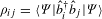. By diagonalizing ρij one obtains ρ(1)(r|r′) = ∑ iρiφiNO(r)φiNO(r′). The eigenvalues ρ1ρ2 ≥ … satisfy ∑ ρi = N and are known as natural occupations, the eigenvectors φiNO(r) as natural orbitals. If there is only one eigenvaluethe BEC is condensed13, if more than onethe BEC is fragmented14. The diagonal ρ(r) ≡ ρ(1)(r|r′ = r) is the single-particle density of the N-boson wavefunction.

### Single-shot algorithm.

Here we show how single shots can be simulated from a general N-boson wavefunction expanded in M orbitals |Ψ〉 = ∑ nCn|n〉, where |n〉 = |n1, …, nM〉 and ∑ i=1Mni = N. The special case M = 2 has been treated in earlier works1,3,4. The goal is to draw the positions r1′, …, rN′ of N bosons from the probability distribution P(r1, …, rN). We achieve this by evaluating the conditional probabilities in (1).

For this purpose we define reduced wavefunctionsof n = Nk bosons with normalization constants. The respective single-particle densities are given byand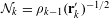. The first position r1′ is drawn randomly from the distribution P(r) = ρ0(r)/N. Assuming that positions rk′, …, r1′ have already been drawn, the conditional probability density for the next particle P(r|rk′, …, r1′) = P(r, rk′, …, r1′)/P(rk′, …, r1′) is given by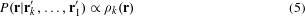because P(rk′, …, r1′) is a constant. The problem is thus reduced to obtaining the wavefunction |Ψ(k)〉 = ∑ nCn(k)|n〉 from the wavefunction |Ψ(k−1)〉 = ∑ nCn(k−1)|n〉, where the sums over run over all configurations of n and n + 1 bosons, respectively. Defining nq = (n1, …, nq + 1, …, nM) one finds from (4) thatUsing (6) in a general M-orbital algorithm requires an ordering of theconfigurations |n〉 for all particle numbers n = 1, …, N. Combinadics11 provide such an ordering by assigning the indexto each configuration |n〉. Using (7) all coefficients Cn(k) can then be obtained by evaluating the sums in (6) andis determined by normalization. Using the coefficients Cn(k) we evaluate ρk(r) and by means of (5) we then draw rk+1′ from P(r|rk′, …, r1′). This concludes the algorithm to simulate single shots. It is now easy to see that also correlation functions of arbitrary order can be evaluated. By realizing thatthe kth order correlation function is evaluated at r1, …, rk as the product of the reduced densities ρj−1(rj). Thus, to evaluate the correlation function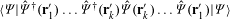the only modification to the single-shot algorithm above consists in choosing the positions r1′, …, rk′ rather than drawing them randomly.

### MCTDHB.

In the MCTDHB (refs 10,11,12) method the many-boson wavefunction is expanded in all configurations that can be constructed by distributing N bosons over M time-dependent orbitals φi(r, t). The ansatz for the time-dependent many-boson wavefunction reads: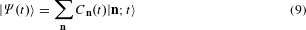In (9) the Cn(t) are time-dependent expansion coefficients and the |n; t〉 are time-dependent permanents built from the orbitals φi(r, t):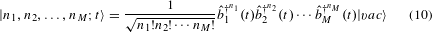The MCTDHB equations of motion are derived by requiring stationarity of the many-body Schrödinger action functional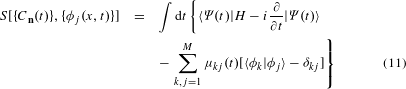with respect to variations of the coefficients and the orbitals. The μkj(t) are time-dependent Lagrange multipliers that ensure the orthonormality of the orbitals. With increasing M the solution of the MCTDHB equations converges to an exact solution of the time-dependent many-body Schrödinger equation and numerically exact results have been obtained previously23,24. For bosons interacting via a delta-function interaction and M = 1, the MCTDHB equations of motion reduce to the time-dependent Gross–Pitaevskii equation. For more information see the literature10,11,12.

### Parameters.

For all D = 2 dimensional simulations in this work we assume tight harmonic confinement with a frequency ωz and a harmonic oscillator length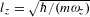along the z-direction. The bosons interact via a 2D regularized contact interaction potential (2λ0/m)δε(r), with δε(r) = (2πε2)−1$e - r 2 / 2 ε 2$, r = (x, y) and a dimensionless interaction strength, where a is the scattering length and m the mass of boson. We note that it is important to regularize contact interaction potentials for D > 1 (refs 25,26). For the collision of attractively interacting BECs the external potential used for the simulations is given by V (r) = Vx(x) + Vg(x) + Vy(y), with Vx(x) = (1/2)x2x2, Vy(y) = (1/2)y2y2, and Vg(x) = $C e - x 2 / 2 σ 2$, where C = 2ωx2. We obtain dimensionless units = m = 1 and the Hamiltonian (2) by measuring energy in units of ωy, length in units of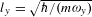and time in units of 1/ωy. We use a plane-wave discrete variable representation to represent all orbitals and operators. The width of the contact interaction is ε = 0.15 and the grid spacing is Δx = Δy = ε/2 unless stated otherwise. For the elongated trap the parameter values are ωx = 0.07, ωy = 1 and σ = 10 on a grid [−43.2,43.2] × [−3.6,3.6], which creates a trap with a flat bottom at the centre. For the rotating BEC the parameter values are ωx = ωy = 1. η(t) is linearly ramped up from zero to ηmax = 0.1 over a time span tr = 80. η(t) is then kept constant for tup = 220 and ramped back down to zero over a time span tr. The potential Vs(r, t) = (1/2)η(t)[x(t)2y(t)2] rotates harmonically with x(t) = xcos(Ωt) + ysin(Ωt) and y(t) = −xsin(Ωt) + ycos(Ωt), where Ω = π/4. The grid size is [−8,8] × [−8,8] with 214 grid points in each direction.

For the D = 1 dimensional simulations we assume tight harmonic confinement along the y- and z-directions with a radial frequency ω = ωy = ωz and an oscillator length. The contact interaction potential is then given by (22a/ml2)δε(x), with δε(x) = (2πε2)−1/2$e - x 2 / 2 ε 2$. We use ω as the unit of energy and l as the unit of length. The dimensionless interaction strength is then given by λ0 = 2a/l. The harmonic potential along the x-direction ωx = 1/100 is much weaker than the radial confinement ω = 1. The grid size is [−90,90].

### Image processing.

The histograms of the positions of particles obtained using the single-shot algorithm have a resolution that is determined by the grid spacing. For better visibility and in analogy to a realistic imaging system we convolved the data points of each histogram with a point-spread function (PSF). As a PSF we used a Gaussian of width 3 × 3 pixels.

## References

1. 1

Javanainen, J. & Yoo, S. M. Quantum phase of a Bose–Einstein condensate with an arbitrary number of atoms. Phys. Rev. Lett. 76, 161–164 (1996).

2. 2

Castin, Y. & Dalibard, J. Relative phase of two Bose–Einstein condensates. Phys. Rev. A 55, 4330–4337 (1997).

3. 3

Dziarmaga, J., Karkuszewski, Z. P. & Sacha, K. Images of the dark soliton in a depleted condensate. J. Phys. B 36, 1217–1229 (2003).

4. 4

Dagnino, D., Barberán, N. & Lewenstein, M. Vortex nucleation in a mesoscopic Bose superfluid and breaking of the parity symmetry. Phys. Rev. A 80, 053611 (2009).

5. 5

Sinatra, A., Lobo, C. & Castin, Y. The truncated Wigner method for Bose-condensed gases: limits of validity and applications. J. Phys. B 35, 3599–3631 (2002).

6. 6

Martin, A. D. & Ruostekoski, J. Quantum and thermal effects of dark solitons in a one-dimensional Bose gas. Phys. Rev. Lett. 104, 194102 (2010).

7. 7

Blakie, P. B., Bradley, A. S., Davis, M. J., Ballagh, R. J. & Gardiner, C. W. Dynamics and statistical mechanics of ultra-cold Bose gases using c-field techniques. Adv. Phys. 57, 363–455 (2008).

8. 8

Polkovnikov, A. Phase space representation of quantum dynamics. Ann. Phys. 325, 1790–1852 (2010).

9. 9

Pollet, L. Recent developments in quantum Monte Carlo simulations with applications for cold gases. Rep. Prog. Phys. 75, 094501 (2012).

10. 10

Alon, O. E., Streltsov, A. I. & Cederbaum, L. S. Multiconfigurational time-dependent Hartree method for bosons: many-body dynamics of bosonic systems. Phys. Rev. A 77, 033613 (2008).

11. 11

Streltsov, A. I., Alon, O. E. & Cederbaum, L. S. General mapping for bosonic and fermionic operators in Fock space. Phys. Rev. A 81, 022124 (2010).

12. 12

Streltsov, A. I., Sakmann, K., Lode, A. U. J., Alon, O. E. & Cederbaum, L. S. The Multiconfigurational Time-Dependent Hartree for Bosons Package Version 2.3 (2013); http://mctdhb.org

13. 13

Penrose, O. & Onsager, L. Bose–Einstein condensation and liquid helium. Phys. Rev. 104, 576–584 (1956).

14. 14

Nozières, P. & James, D. S. Particle vs. pair condensation in attractive Bose-liquids. J. Phys. France 43, 1133–1148 (1982).

15. 15

Parker, N. G., Martin, A. M., Cornish, S. L. & Adams, C. S. Collisions of bright solitary matter waves. J. Phys. B 41, 045303 (2008).

16. 16

Streltsov, A. I., Alon, O. E. & Cederbaum, L. S. Swift loss of coherence of soliton trains in attractive Bose–Einstein condensates. Phys. Rev. Lett. 106, 240401 (2011).

17. 17

Mullin, W. J. & Laloë, F. Interference of Bose–Einstein condensates: quantum nonlocal effects. Phys. Rev. A 78, 061605 (2008).

18. 18

Nguyen, J. H. V., Dyke, P., Luo, D., Malomed, B. A. & Hulet, R. G. Collisions of matter-wave solitons. Nature Phys. 10, 918–922 (2014).

19. 19

Fetter, A. L. Rotating trapped Bose–Einstein condensates. Rev. Mod. Phys. 81, 647–691 (2009).

20. 20

Dagnino, D., Barberán, N., Lewenstein, M. & Dalibard, J. Vortex nucleation as a case study of symmetry breaking in quantum systems. Nature Phys. 5, 431–437 (2009).

21. 21

Weiner, S. E., Tsatsos, M. C., Cederbaum, L. S. & Lode, A. U. J. Angular momentum in interacting many-body systems hides in phantom vortices. Preprint at http://arXiv.org/abs/1409.7670

22. 22

Brey, L., Johnson, N. F. & Halperin, B. I. Optical and magneto-optical absorption in parabolic quantum wells. Phys. Rev. B 40, 10647–10649 (1989).

23. 23

Sakmann, K., Streltsov, A. I., Alon, O. E. & Cederbaum, L. S. Exact quantum dynamics of a Bosonic Josephson junction. Phys. Rev. Lett. 103, 220601 (2009).

24. 24

Lode, A. U. J., Sakmann, K., Alon, O. E., Cederbaum, L. S. & Streltsov, A. I. Numerically exact quantum dynamics of bosons with time-dependent interactions of harmonic type. Phys. Rev. A 86, 063606 (2012).

25. 25

Esry, B. D. & Greene, C. H. Validity of the shape-independent approximation for Bose–Einstein condensates. Phys. Rev. A 60, 1451–1462 (1999).

26. 26

Doganov, R. A., Klaiman, S., Alon, O. E., Streltsov, A. I. & Cederbaum, L. S. Two trapped particles interacting by a finite-range two-body potential in two spatial dimensions. Phys. Rev. A 87, 033631 (2013).

## Acknowledgements

K.S. acknowledges financial support through the Karel Urbanek Postdoctoral Research Fellowship. Computing time was provided by the High Performance Computing Center (HLRS) in Stuttgart, Germany. We would like to thank J. Schmiedmayer for interesting discussions about partially destructive measurements.

## Author information

Authors

### Contributions

K.S. and M.K. conceived the ideas and designed the study. K.S. developed the algorithm and carried out the simulations. K.S. and M.K. wrote the paper.

### Corresponding author

Correspondence to Kaspar Sakmann.

## Ethics declarations

### Competing interests

The authors declare no competing financial interests.

## Supplementary information

### Supplementary information

Supplementary information (PDF 5371 kb)

## Rights and permissions

Reprints and Permissions

• ### Colloquium : Multiconfigurational time-dependent Hartree approaches for indistinguishable particles

• Axel U. J. Lode
• , Camille Lévêque
• , Alexej I. Streltsov
•  & Ofir E. Alon

Reviews of Modern Physics (2020)

• ### Relaxation of Shannon entropy for trapped interacting bosons with dipolar interactions

• Sangita Bera
• , Sudip Kumar Haldar
• , Barnali Chakrabarti
• , Andrea Trombettoni
•  & V. K. B. Kota

The European Physical Journal D (2020)

• ### MCTDH-X: The multiconfigurational time-dependent Hartree method for indistinguishable particles software

• Rui Lin
• , Paolo Molignini
• , Luca Papariello
• , Marios C Tsatsos
• , Camille Lévêque
• , Storm E Weiner
• , Elke Fasshauer
• , R Chitra
•  & Axel U J Lode

Quantum Science and Technology (2020)

• ### Unconventional pairing in one-dimensional systems of a few mass-imbalanced ultracold fermions

• Patrycja Łydżba
•  & Tomasz Sowiński

Physical Review A (2020)

• ### Ground state properties of trapped boson system with finite-range Gaussian repulsion: exact diagonalization study

• Mohd Imran
•  & M A H Ahsan

Journal of Physics B: Atomic, Molecular and Optical Physics (2020)

## Search